# DAV Class 3 Maths Chapter 4 Worksheet 1 Solutions

The DAV Class 3 Maths Book Solutions and DAV Class 3 Maths Chapter 4 Worksheet 1 Solutions of Multiplication offer comprehensive answers to textbook questions.

## DAV Class 3 Maths Ch 4 WS 1 Solutions

Multiply.

Question 1.Solution:
366

Question 2.Solution:
696

Question 3.Solution:
844Question 4.Solution:
686

Question 5.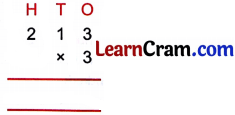Solution:
639

Question 6.Solution:
666Question 7.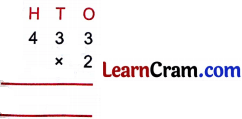Solution:
866

Question 8.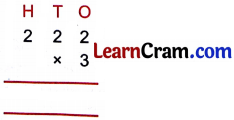Solution:
666

Question 9.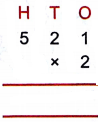Solution:
1042### DAV Class 3 Maths Chapter 4 Worksheet 1 Notes

Multiplication is the repeated addition of a number. It is shown by the sign ‘×’. The result of multiplication is called the product.
5 × 3 = 15
So, 15 is the product of 5 and 3. We say 5 and 3 are factors of 15.

0 × 0 × 0 × 0 × 0 = 0
5 × 0 = 0
0 × 5 = 0
Number × 0 = 0
Number × 1 = Same Number
1 × Number = Same number

Multiplication Tables (1 – 15)

1 × 1 =1
1 × 2 = 2
1 × 3 = 3
1 × 4 = 4
1 × 5 = 5
1 × 6 = 6
1 × 7 = 7
1 × 8= 8
1 × 9 = 9
1 × 10 = 10

2 × 1 = 2
2 × 2 = 4
2 × 3 = 6
2 × 4 = 8
2 × 5 = 10
2 × 6 = 12
2 × 7 = 14
2 × 8 = 16
2 × 9 = 18
2 × 10 = 20

3 × 1 = 3
3 × 2 = 6
3 × 3 = 9
3 × 4 = 12
3 × 5 = 15
3 × 6 = 18
3 × 7 = 21
3 × 8 = 24
3 × 9 = 27
3 × 10 = 304 × 1 = 4
4 × 2 = 8
4 × 3 = 12
4 × 4 = 16
4 × 5 = 20
4 × 6 = 24
4 × 7 = 28
4 × 8 = 32
4 × 9 = 36
4 × 10 = 40

5 × 1 = 5
5 × 2 = 10
5 × 3 = 15
5 × 4 = 20
5 × 5 = 25
5 × 6 = 30
5 × 7 = 35
5 × 8 = 40
5 × 9 = 45
5 × 10 = 50

6 × 1 = 6
6 × 2 = 12
6 × 3 = 18
6 × 4 = 24
6 × 5 = 30
6 × 6 = 36
6 × 7 = 42
6 × 8 = 48
6 × 9 = 54
6 × 10 = 607 × 1 = 7
7 × 2 = 14
7 × 3 = 21
7 × 4 = 28
7 × 5 = 35
7 × 6 = 42
7 × 7 = 49
7 × 8 = 56
7 × 9 = 63
7 × 10 = 70

8 × 1 = 8
8 × 2 = 16
8 × 3 = 24
8 × 4 = 32
8 × 5 = 40
8 × 6 = 48
8 × 7 = 56
8 × 8 = 64
8 × 9 = 72
8 × 10 = 80

9 × 1 = 9
9 × 2 = 18
9 × 3 = 27
9 × 4 = 36
9 × 5 = 45
9 × 6 = 54
9 × 7 = 63
9 × 8 = 72
9 × 9 = 81
9 × 10 = 1010 × 1 = 10
10 × 2 = 20
10 × 3 = 30
10 × 4 = 40
10 × 5 = 50
10 × 6 = 60
10 × 7 = 70
10 × 8 = 80
10 × 9 = 90
10 × 10 = 100

1 × 1 = 11
11 × 2 = 22
11 × 3 = 33
11 × 4 = 44
11 × 5 = 55
11 × 6 = 66
11 × 7 = 77
11 × 8 = 88
11 × 9 = 99
11 × 10 = 110

12 × 1 = 12
12 × 2 = 24
12 × 3 = 36
12 × 4 = 48
12 × 5 = 60
12 × 6 = 72
12 × 7 = 84
12 × 8 = 96
12 × 9 = 108
12 × 10 = 12013 × 1 = 13
13 × 2 = 26
13 × 3 = 39
13 × 4 = 52
13 × 5 = 65
13 × 6 = 78
13 × 7 = 91
13 × 8 = 104
13 × 9 = 117
13 × 10 = 130

14 × 1 = 14
14 × 2 = 28
14 × 3 = 42
14 × 4 = 56
14 × 5 = 70
14 × 6 = 84
14 × 7 = 98
14 × 8= 112
14 × 9 = 126
14 × 10 = 140

15 × 1 = 15
15 × 2 = 30
15 × 3 = 45
15 × 4 = 60
15 × 5 = 75
15 × 6 = 90
15 × 7 = 105
15 × 8 = 120
15 × 9 = 135
15 × 10 = 150Multiplication (3 – digits by 1 – digit)First multiply ones by 2 then tens by 2 and lastly hundreds by 2.

Let us have some fun with Multiplication

1.Each flower has 5 petals.
There are ______ petals in 9 such flowers.
Solution:
45

2.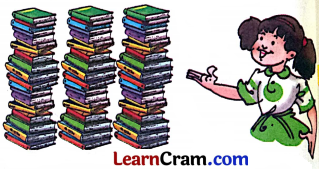Each bundle has 20 books.
There are ______ books in 3 such bundles.
Solution:
60

3.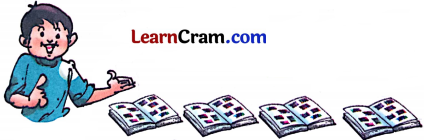I have pasted 40 stamps in one scrap file.
I have pasted ______ stamps in 4 such scrap files.
Solution:
1604.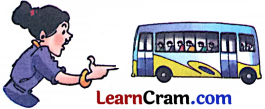One bus can carry 62 people.
3 such buses can carry ______ people.
Solution:
186

5.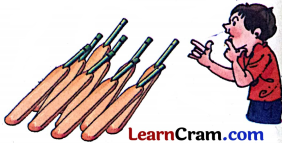Cost of one bat is ₹ 70.
9 such bats will cost ₹ ______.
Solution:
₹ 630